## Rational Exponents

If n is a natural number greater than 1 and b is any real number, then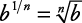and since a negative exponent indicates a reciprocal, then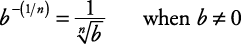##### Example 1

Simplify each of the following.

1. 36 1/2

2. (72 x 4 y) 1/3

3. 16 –(1/4)

1.2.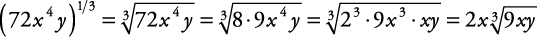3.If n is a natural number greater than 1, m is an integer, and b is a non‐negative real number, then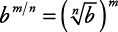and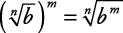##### Example 2

Simplify each of the following.

1. 27 2/3

2. 25 –(3/2)

1.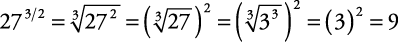2.Back to Top
A18ACD436D5A3997E3DA2573E3FD792A